@Dounm 2018-08-26T03:28:37.000000Z 字数 19699 阅读 33195

# Word2Vec-知其然知其所以然

Blog MachineLearning

Word2vec的模型很简单，训练也很简单。但是针对为什么模型结构这么设计？为什么采用Hierarchical Softmax的框架？或是为什么采用Negative Sampling的框架？网上貌似没有一个比较好的介绍。

## 1 介绍

Word2vec的模型以大规模语料库作为输入，然后生成一个向量空间（通常为几百维）。词典中的每个词都对应了向量空间中的一个独一的向量，而且语料库中拥有共同上下文的词映射到向量空间中的距离会更近

## 2 背景知识

### 2.1 Huffman树与Huffman编码

#### 2.1.1 Huffman树的概念

• 路径/路径长度：一棵树上一个点到另一个节点的通路称为路径，路径上分支（边）的个数称为路径长度。路径长度通常都是针对根节点而言，因此，设根节点层数为1，则从根节点到$L$层节点路径长度为$L-1$.
• 结点的权/带权路径长度：为树中的某个节点赋予一个值，则该值就为该节点的权。带权路径长度指的是：从根节点到该节点之间的路径长度与该节点权的乘积。
• 树的带权路径长度：树的所有叶子节点的带权路径长度之和。
• Huffman树（霍夫曼树/最优二叉树）：给定n个权值作为n个叶子节点，则带权路径最小的树称为Huffman树。

#### 2.1.2 Huffman树的构造

1. ${w_1,w_2,\dots,w_3}$看成是有$n$棵树的森林（每棵树仅有一个节点）
2. 从森林中选择两个根节点权值最小的树合并，作为一棵新树的左右子树，且新树的根节点权值为其左右子树根节点权值之和
3. 从森林中删除被选中的两棵树，并且将新树加入森林
4. 重复2-3步，知道森林中只有一棵树为止，则该树即所求的Huffman树

#### 2.1.3 Huffman树构造算法理解与证明

Huffman树构造算法的理解大概就是这样，具体的形式化证明请参见『算法导论16.3节』。

#### 2.1.4 Huffman编码

Word2vec算法也用了Huffman编码，它把训练语料中的词当成叶子节点，其在语料中出现的次数当做权值，通过构造响应的Huffman树来对每一个词进行Huffman编码。

### 2.2 N-gram模型

#### 2.2.3 N-gram模型的实践

• 计算复杂度：由于参数的个数是$N+N^2+\dots+N^n$个，因此很明显，$n$越大，计算复杂度越大，而且是指数级增大。
• 模型效果：理论上是$n$越大越好，但是$n$越大的时候，模型效果提升幅度就会越小。例如，但$n$从3到4的效果提升可能就远比不上从2到3的效果提升。

### 2.3 概率模型函数化

N-gram模型是存储下来所有可能的概率参数，然后计算时对概率进行连乘。

• $C$是语料库(Corpus)
• $Context(w)$是词$w$的上下文(Context)。对于N-gram模型来说，$Context(w_i)=w_{i-n+1}^{i-1}$

### 2.4 神经概率语言模型

#### 2.4.1 词向量

• One-hot Representation：用维度为字典长度的向量表示一个词，仅一个分量为1，其余为0。缺点是容易收到维度灾难的困扰，而且不能很好的刻画词与词之间的关系。
• Distributed Representation：每个词映射为固定长度的短向量。通过刻画两个向量之间的距离来刻画两个向量之间的相似度。

#### 2.4.2 神经概率语言模型的网络结构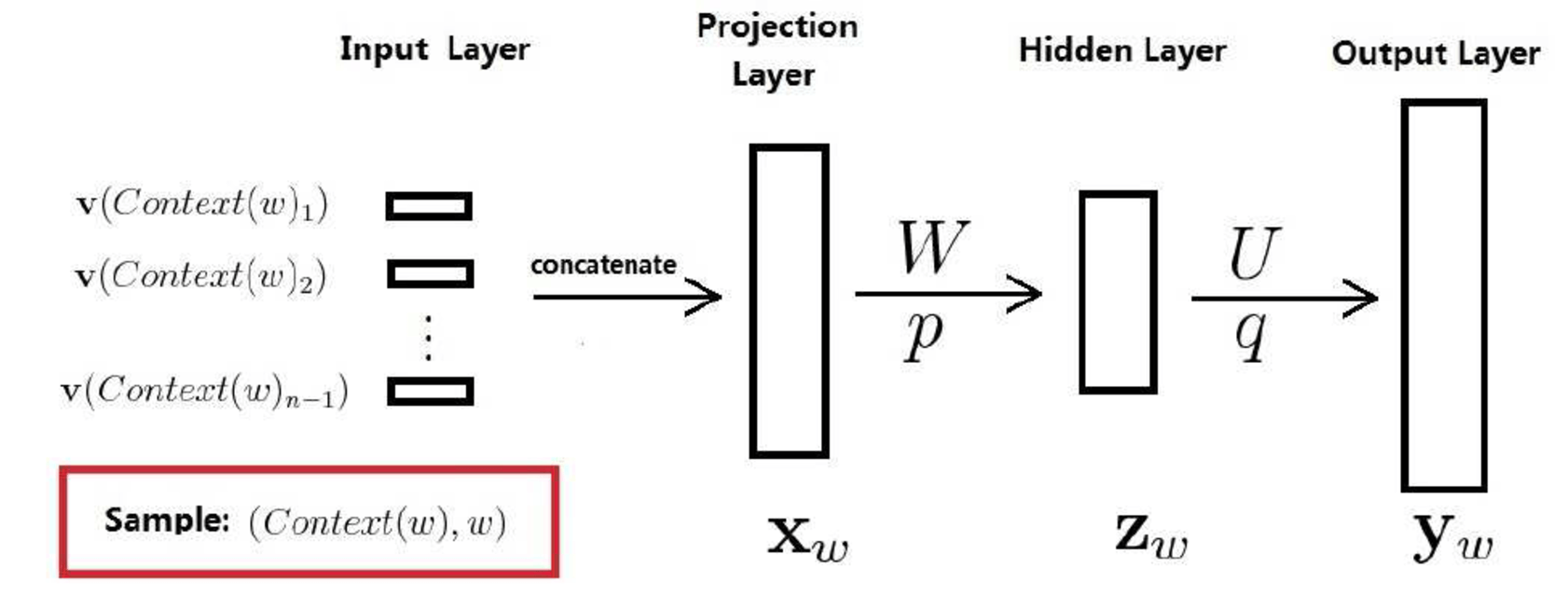• 训练样本$(Content(w),w)$$w$是语料C中的每一个词，$Content(w)$取为其前面$n-1$个词
• 投影层向量$X_w$：将该训练样本$(Content(w),w)$的前$n-1$个词的『词向量首尾相接』拼接在一起构成$X_w$$X_w$长度为$(n-1)\cdot m$$m$即词向量长度
• 隐藏层向量$Z_w$
• 输出层向量$y_w$：维度为$N=|D|$,即词典$D$中词的个数。

#### 2.4.3 神经概率语言模型的优缺点

1. 词语与词语间的相似度可以通过词向量来体现
2. 基于词向量的模型自带『平滑化』功能，无需额外处理。因为根据公式$\eqref{eq:3}$ $p(w|Context(w))$不可能为0。

• 投影层节点数为上下文词数量*词向量维度。上下文数量通常不超过$5$，词向量维度在$10^2$量级，
• 隐层节点数在$10^2$量级，
• 输出层节点数为词典大小，在$10^5$量级。

## 3 【重要】Word2vec相比于神经概率语言模型的改进

### 3.1 优化网络结构

Word2vec的网络机构相对于神经概率语言模型简化了很多，但是正是因为模型简单，因此训练起来更加容易，就能训练更多的数据。而神经概率语言模型结构复杂，导致训练较慢，难以训练更多的数据。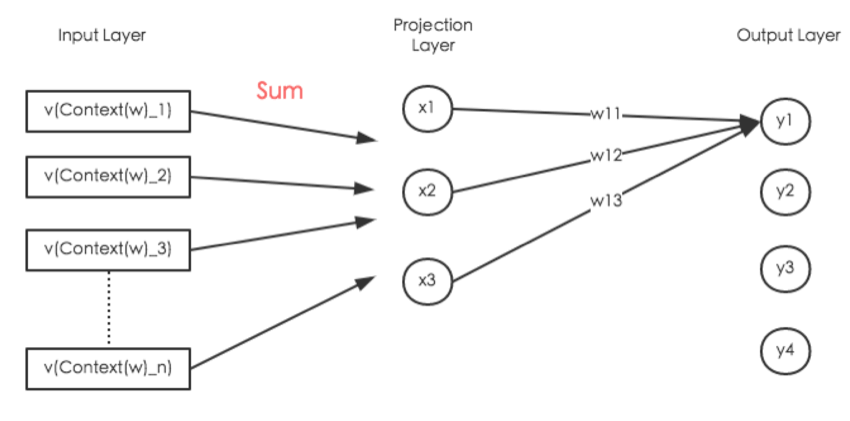（Output Layer和Projection Layer之间的连线没有画完）

### 3.2 优化Softmax归一化

#### 3.2.1 使用Hierarchical Softmax优化

A computationally efficient approximation of the full softmax is the hierarchical softmax.

Hierarchical softmax采用的树是二叉树。它将树上的叶子节点分配给词典里的词，而将从树根到叶子节点的路径上的每个非叶子结点都看作是二分类，路径上的二分类概率连乘的结果就是该叶子节点对应的词的概率。

#### 3.2.2 使用Negative Sampling优化

NCE posits that a good model should be able to differentiate data from noise by means of logistic regression.

Word2vec采用的Negative Sampling是NCE的一种简化版本，目的是为了提高训练速度以及改善所得词的质量。相比于Hierarchical Softmax，Negative Sampling不再采用Huffman树，而是采用随机负采样

Negative Sampling简单理解起来的话，是这样的。

## 4 基于Hierarchical Softmax的模型

Word2vec中的两个重要模型是：CBOW模型（Continuous Bag-of-Words Model）和Skip-gram模型（Continuous Skip-gram模型）。对于这两个模型，Word2vec给出了两套框架，分别是基于Hierarchical Softmax和Negative Sampling来设计的。本节先介绍Hierarchical Softmax框架。

### 4.1 CBOW模型

CBOW模型全名为$Continous \;bag-of-words$。之所以叫$bag-of-words$是因为输入层到投影层的操作由『拼接』变成了『叠加』，对于『叠加而言』，无所谓词的顺序，所以称为$词袋，bag-of-words$模型。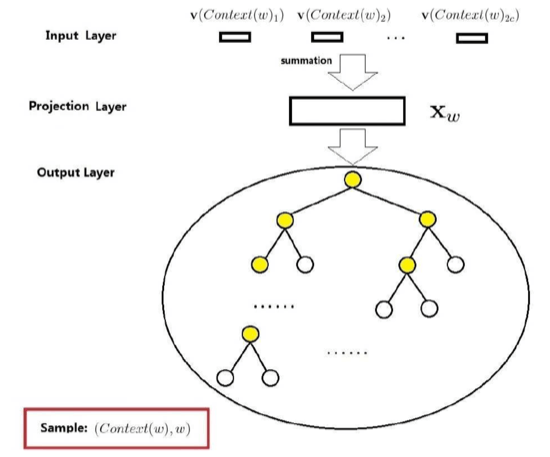• 上下文$Context(w)$：由$w$前后各$c$个词构成
• 输入层：包含$2c$个词的词向量
• 投影层：讲输入层的$2c$个词做『累加求和』。
• 输出层：输出层对应的是一棵Huffman树。该Huffman树以语料中出现的词为叶子节点，以各词在语料中出现的次数当做权值构造出来的。该树中，叶子节点总共有$N$个（$N=|D|$）。

• 输入层到投影层：CBOW是『累加』，神经概率语言是『拼接』
• 隐藏层：CBOW无隐藏层，神经概率语言有
• 输出层：CBOW是『树形结构』，神经概率语言是『线性结构』

#### 4.1.1 梯度计算

• $p^w$：从根节点到$w$对应的叶子节点的路径
• $l^w$：路径$p^w$上包含的节点的个数
• $p_1^w,p_2^w,\dots,p_{l^w}^w$：路径$p^w$上对应的节点
• $d_2^w,d_3^w,\dots,d_{l^w}^w\in \{0,1\}$：路径上的节点对应的Huffman编码，根节点不对应编码
• $\theta_1^w,\theta_2^w,\dots,\theta_{l^w}^w\in \mathbb{R}^m$：路径上的『非叶子节点』对应的词向量

• 分为正类的概率：$\sigma(X_w^T\theta)=\frac{1}{1+e^{-X_w^T\theta}}$
• 分为负类的概率：$1-\sigma(X_w^T\theta)$

$\mathcal{L}(w,j)$等于如下内容：

#### 4.1.2 伪代码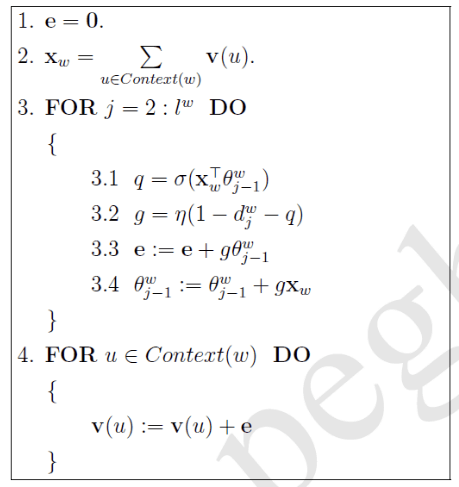### 4.2 Skip-gram模型

Skip-gram模型是已知当前词$w$，对其上下文$Context(w)$中的词进行预测。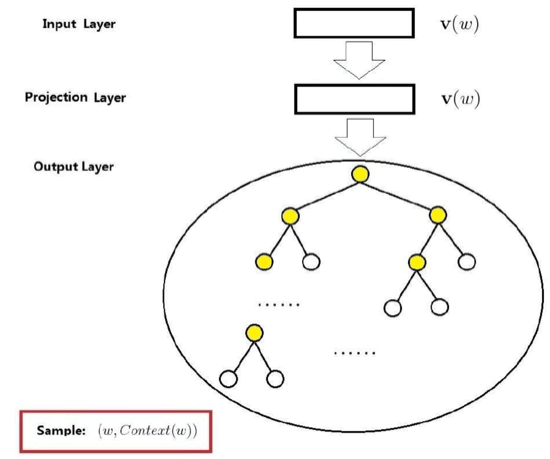• 输入层：当前样本的中心词$w$的词向量
• 投影层：其实没什么作用
• 输出层：类似CBOW模型，也是一颗Huffman树

#### 4.2.1 梯度计算

$\mathcal{L}(w,u,j)$等于如下内容：

#### 4.2.2 伪代码

skip-gram的伪代码如下：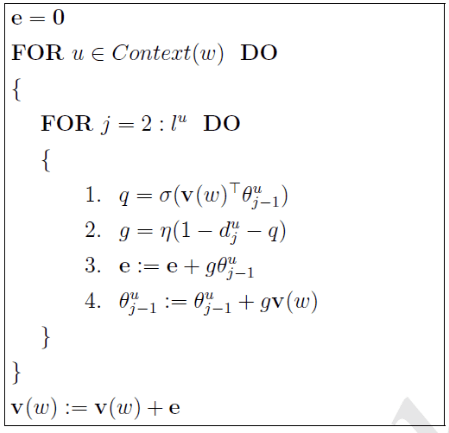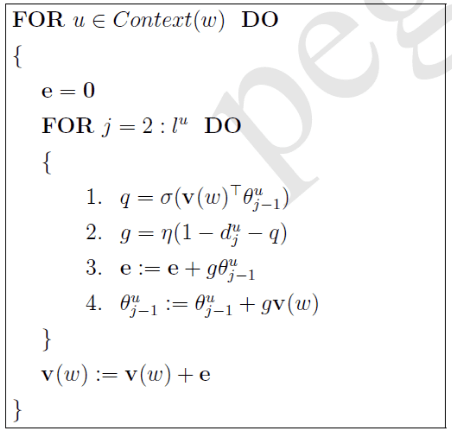## 5 基于Negative Sampling的模型

### 5.2 CBOW模型

CBOW模型中，我们是已知词$w$的上下文$Context(w)$，需要预测$w$

#### 5.2.1 梯度计算

$\mathcal{L}(w,u)$等于如下式子：

#### 5.2.2 伪代码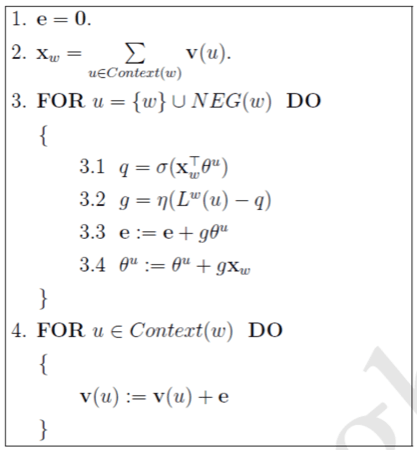## 6 总结

### 6.1 残留问题

• 为什么求最大似然就可以得到较好的词向量？
• 为什么所有的二分类采用的都是逻辑回归，都是Sigmoid函数？

## 7 X2Vec

### 7.1 Paragraph2Vec

Paragraph2Vec的模型中，这个Paragraph Vectors的被训练出来的主要目的是用来预测paragraph中的word。其结构图如下：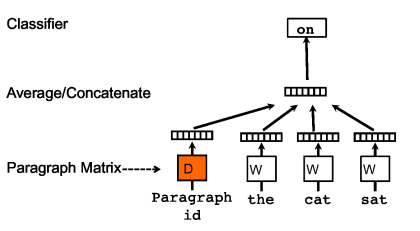### 7.2 推荐系统中的X2Vec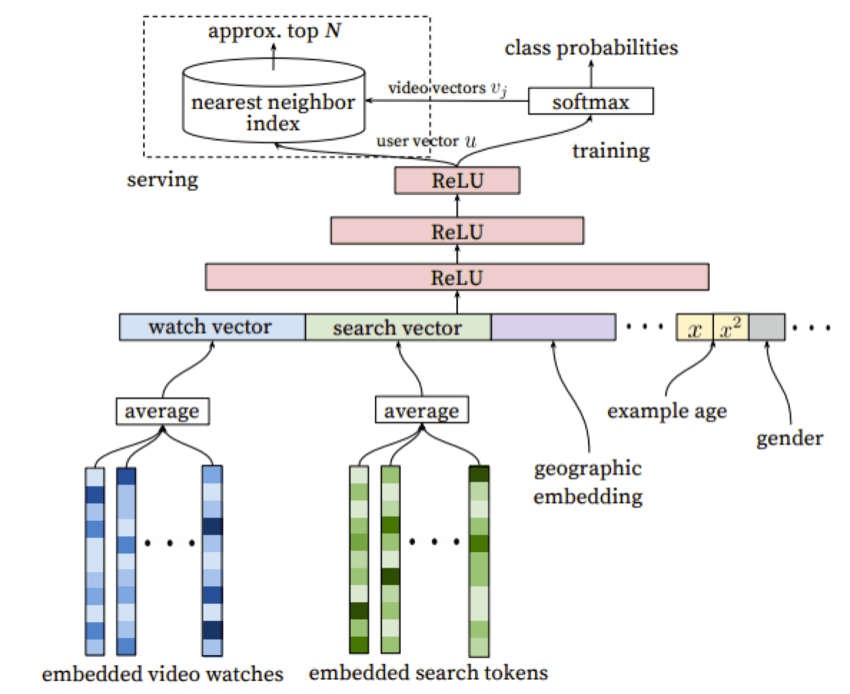We pose recommendation as extreme multiclass classification where the prediction problem becomes accurately classifing a specific video watch $w_t$ at time $t$ among millions of videos $i$ (classes) from a corpus $V$ based on a user $U$ and context $C$.

## 7 参考资料

1. word2vec中的数学原理详解
2. Mikolov T, Chen K, Corrado G, et al. Efficient estimation of word representations in vector space[J]. arXiv preprint arXiv:1301.3781, 2013.
3. Mikolov T, Sutskever I, Chen K, et al. Distributed representations of words and phrases and their compositionality[C]//Advances in neural information processing systems. 2013: 3111-3119.
4. Goldberg Y, Levy O. word2vec Explained: deriving Mikolov et al.'s negative-sampling word-embedding method[J]. arXiv preprint arXiv:1402.3722, 2014.
5. word2vec: negative sampling (in layman term)?
6. Le Q V, Mikolov T. Distributed Representations of Sentences and Documents[C]//ICML. 2014, 14: 1188-1196.
7. 语义分析的一些方法(二)
8. Covington P, Adams J, Sargin E. Deep neural networks for youtube recommendations[C]//Proceedings of the 10th ACM Conference on Recommender Systems. ACM, 2016: 191-198.

 Mikolov T, Sutskever I, Chen K, et al. Distributed representations of words and phrases and their compositionality[C]//Advances in neural information processing systems. 2013: 3111-3119.• 私有
• 公开
• 删除